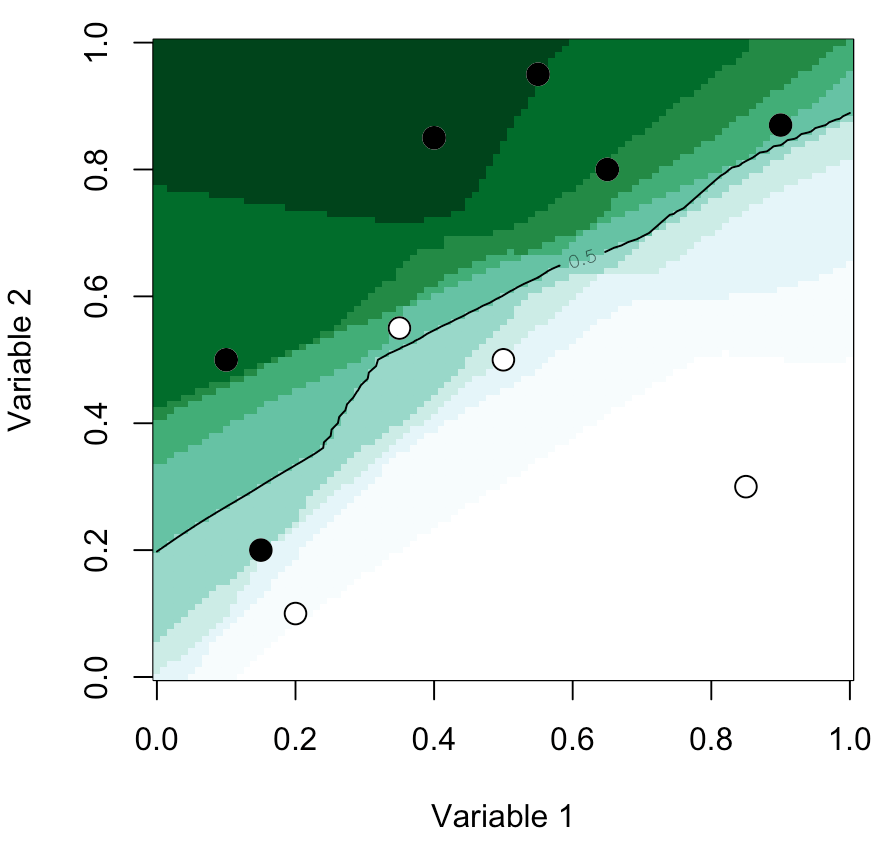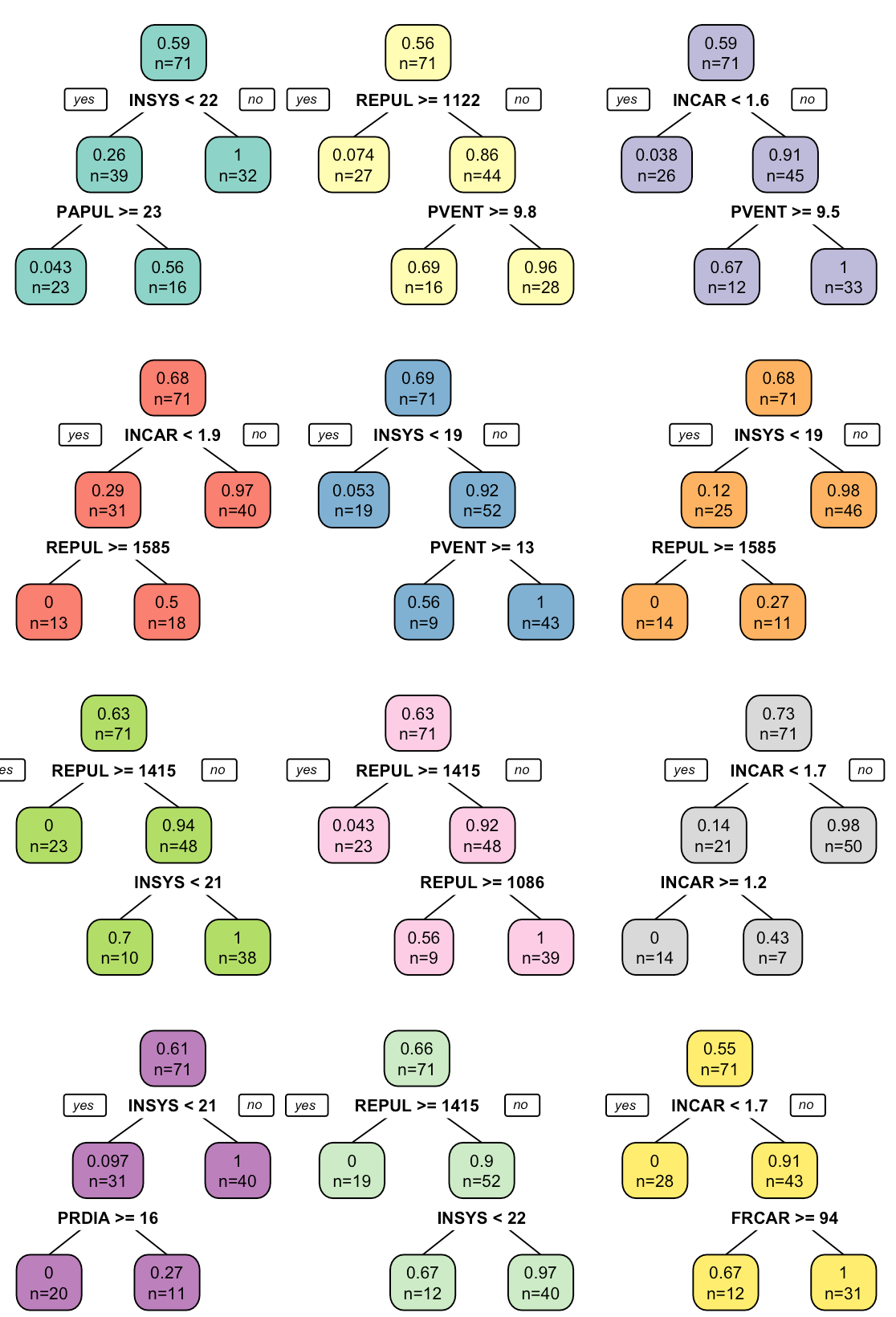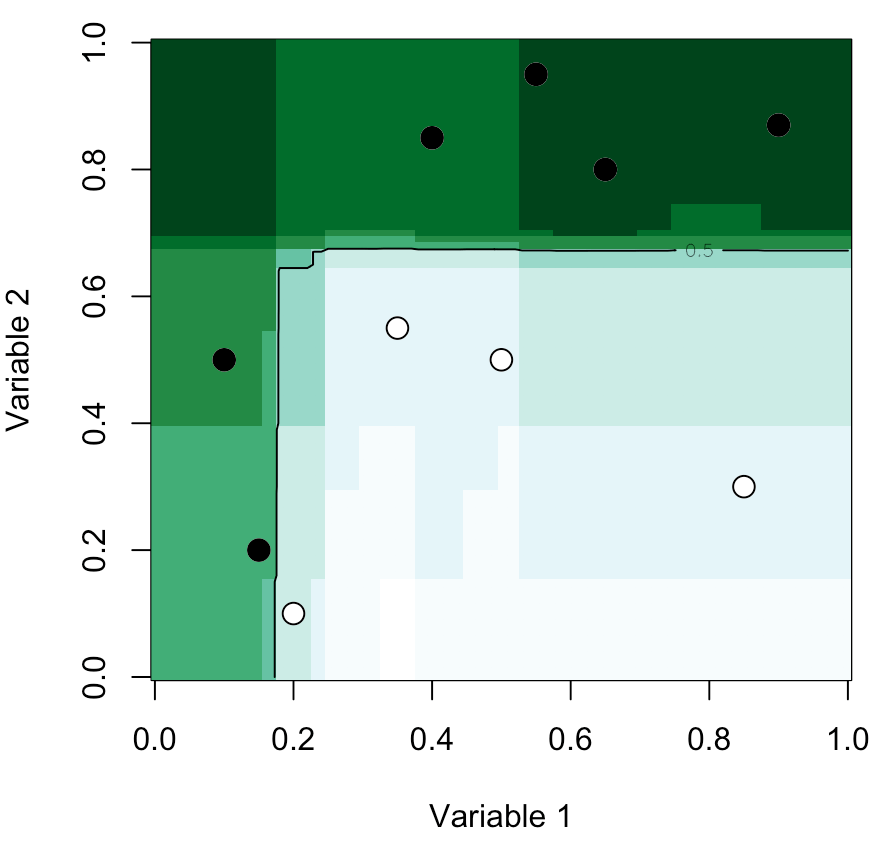Want to share your content on R-bloggers? click here if you have a blog, or here if you don't.

Tenth post of our series on classification from scratch. Today, we’ll see the heuristics of the algorithm inside bagging techniques.

Often, bagging is associated with trees, to generate forests. But actually, it is possible using bagging for any kind of model. Recall that bagging means “boostrap aggregation”. So, consider a model $m:\mathcal{X}\rightarrow \mathcal{Y}$. Let $\widehat{m}_{S}$ denote the estimator of $m$ obtained from sample $S=\{y_i,\mathbf{x}_i\}$ with $i=\{1,\cdots,n\}$.

Consider now some boostrap sample, $S_b=\{y_i,\mathbf{x}_i\}$ with $i$ is randomly drawn from $\{1,\cdots,n\}$ (with replacement). Based on that sample, estimate $\widehat{m}_{S_b}$. Then draw many samples, and consider the agregation of the estimators obtained, using either a majority rule, or using the average of probabilities (if a probabilist model was considered). Hence[latex display=”true”]\widehat{m}^{bag}(\mathbf{x})=\frac{1}{B}\sum_{b=1}^B \widehat{m}_{S_b}(\mathbf{x})[/latex]

## Bagging logistic regression #1

Consider the case of the logistic regression. To generate a bootstrap sample, it is natural to use the technique describe above. I.e. draw pairs $(y_i,\mathbf{x}_i)$ randomly, uniformly (with probability $1/n$) with replacement. Consider here the small dataset, just to visualize. For the b part of bagging, use the following code

L_logit = list()
n = nrow(df)
for(s in 1:1000){
df_s = df[sample(1:n,size=n,replace=TRUE),]
L_logit[[s]] = glm(y~., df_s, family=binomial)}

Then we should aggregate over the 1000 models, to get the agg part of bagging,

p = function(x){
nd=data.frame(x1=x, x2=x)
unlist(lapply(1:1000,function(z) predict(L_logit[[z]],newdata=nd,type="response")))}

We now have a prediction for any new observation

vu = seq(0,1,length=101)
vv = outer(vu,vu,Vectorize(function(x,y) mean(p(c(x,y)))))
image(vu,vu,vv,xlab="Variable 1",ylab="Variable 2",col=clr10,breaks=(0:10)/10)
points(df$x1,df$x2,pch=19,cex=1.5,col="white")
points(df$x1,df$x2,pch=c(1,19)[1+(df$y=="1")],cex=1.5) contour(vu,vu,vv,levels = .5,add=TRUE)## Bagging logistic regression #2 Another technique that can be used to generate a bootstrap sample is to keep all $\mathbf{x}_i$‘s, but for each of them, to draw (randomly) a value for $y$, with[latex display=”true”]Y_{i,b}\sim\mathcal{B}(\widehat{m}_{S}(\mathbf{x}_i))[/latex]since[latex display=”true”]\widehat{m}(\mathbf{x})=\mathbb{P}[Y=1|\mathbf{X}=\mathbf{x}].[/latex]Thus, the code for the b part of bagging algorithm is now L_logit = list() n = nrow(df) reg = glm(y~x1+x2, df, family=binomial) for(s in 1:100){ df_s = df df_s$y = factor(rbinom(n,size=1,prob=predict(reg,type="response")),labels=0:1)
L_logit[[s]] = glm(y~., df_s, family=binomial)
}

The agg part of bagging algorithm remains unchanged. Here we obtain

vu = seq(0,1,length=101)
vv = outer(vu,vu,Vectorize(function(x,y) mean(p(c(x,y)))))
image(vu,vu,vv,xlab="Variable 1",ylab="Variable 2",col=clr10,breaks=(0:10)/10)
points(df$x1,df$x2,pch=19,cex=1.5,col="white")
points(df$x1,df$x2,pch=c(1,19)[1+(df$y=="1")],cex=1.5) contour(vu,vu,vv,levels = .5,add=TRUE)Of course, we can use that code we check the prediction obtain on the observations we have in our sample. Just to change, consider here the myocarde data. The entiere code is here L_logit = list() reg = glm(as.factor(PRONO)~., myocarde, family=binomial) for(s in 1:1000){ myocarde_s = myocarde myocarde_s$PRONO = 1*rbinom(n,size=1,prob=predict(reg,type="response"))
L_logit[[s]] = glm(as.factor(PRONO)~., myocarde_s, family=binomial)
}
p = function(x){
nd=data.frame(FRCAR=x, INCAR=x, INSYS=x, PRDIA=x,
PAPUL=x, PVENT=x, REPUL=x)
unlist(lapply(1:1000,function(z) predict(L_logit[[z]],newdata=nd,type="response")))}

For the first observation, with our 1000 simulated datasets, and our 1000 models, we obtained the following estimation for the probability to die.

histo = function(i){
x = as.numeric(myocarde[i,1:7])
v_x = p(x)
hist(v_x,proba=TRUE,breaks=seq(0,1,by=.05),xlab="",main="",
col=rep(c(rgb(0,0,1,.4),rgb(1,0,0,.4)),each=10),ylim=c(0,5))
segments(mean(v_x),0,mean(v_x),5,col="red",lty=2)
points(myocarde$PRONO[i],0,pch=19,cex=2) xi = round(mean(v_x.5)*1000)/10 text(.75,-.1,paste(xi,"%",sep=""),col=rgb(1,0,0,.6))} histo(1) histo(4) Hence, for the first observation, in 77.8% of the models, the predicted probability was higher than 50%, and the average probability was actually close to 75%.or, for observation 22, predictions very close to the first one (except that the first one died, while the 22nd survived) histo(23) histo(11) and, we observe here## Bagging trees Let’s now get back on our trees, mentioned in the previous post. Bagging was introduced in 1994 by Leo Breiman in Bagging Predictors. If the first section describes the procedure, the second one introduces “Bagging Classification Trees”. Trees are nice for interpretation, but most of the time, they are rather poor predictors. The idea of bagging was to improve the accuracy of classification trees. The idea of bagging to to generate a lot of trees clr12 = c("#8dd3c7","#ffffb3","#bebada","#fb8072","#80b1d3","#fdb462","#b3de69","#fccde5","#d9d9d9","#bc80bd","#ccebc5","#ffed6f") n = nrow(myocarde) par(mfrow=c(4,3)) sed=c(1,2,4,5,6,10,11,21,22,24,27,28,30) for(i in 1:12){ set.seed(sed[i]) idx = sample(1:n, size=n, replace=TRUE) cart = rpart(PRONO~., myocarde[idx,]) prp(cart,type=2,extra=1,box.col=clr12[i])}The strategie is actually the same as before. For the bootstrap part, store the tree in a list L_tree = list() for(s in 1:1000){ idx = sample(1:n, size=n, replace=TRUE) L_tree[[s]] = rpart(as.factor(PRONO)~., myocarde[idx,]) } and for the aggregation part, just take the average of predicted probabilities p = function(x){ nd=data.frame(FRCAR=x, INCAR=x, INSYS=x, PRDIA=x, PAPUL=x, PVENT=x, REPUL=x) unlist(lapply(1:1000,function(z) predict(L_tree[[z]],newdata=nd,type="prob")[,2]))} Because with this example, we cannot visualize predictions, let us run the same code on the smaller dataset L_tree = list() n = nrow(df) for(s in 1:1000){ idx = sample(1:n, size=n, replace=TRUE) L_tree[[s]] = rpart(y~x1+x2, df[idx,],control = rpart.control(cp = 0.25, minsplit = 2)) } p = function(x){ nd=data.frame(x1=x, x2=x) unlist(lapply(1:1000,function(z) predict(L_tree[[z]],newdata=nd,type="prob")[,2]))} vu=seq(0,1,length=101) vv=outer(vu,vu,Vectorize(function(x,y) mean(p(c(x,y))))) image(vu,vu,vv,xlab="Variable 1",ylab="Variable 2",col=clr10,breaks=(0:10)/10) points(df$x1,df$x2,pch=19,cex=1.5,col="white") points(df$x1,df$x2,pch=c(1,19)[1+(df$y=="1")],cex=1.5)
contour(vu,vu,vv,levels = .5,add=TRUE)## Fronm bags to forest

Here, we grew a lot of trees, but it is not stricto sensus a random forest algorithm, as introduced in 1995, in Random decision forests. Actually, the difference is in the creation of decision trees. To understand what happens, get back to the previous post on classification trees. As we’ve seen, when we have a node, we look at possible splits : we consider all possible variable, and all possible threshold. The startegy here will be to draw randomly $k$ variables out of $p$ (with of course [latex]k

To be continued…